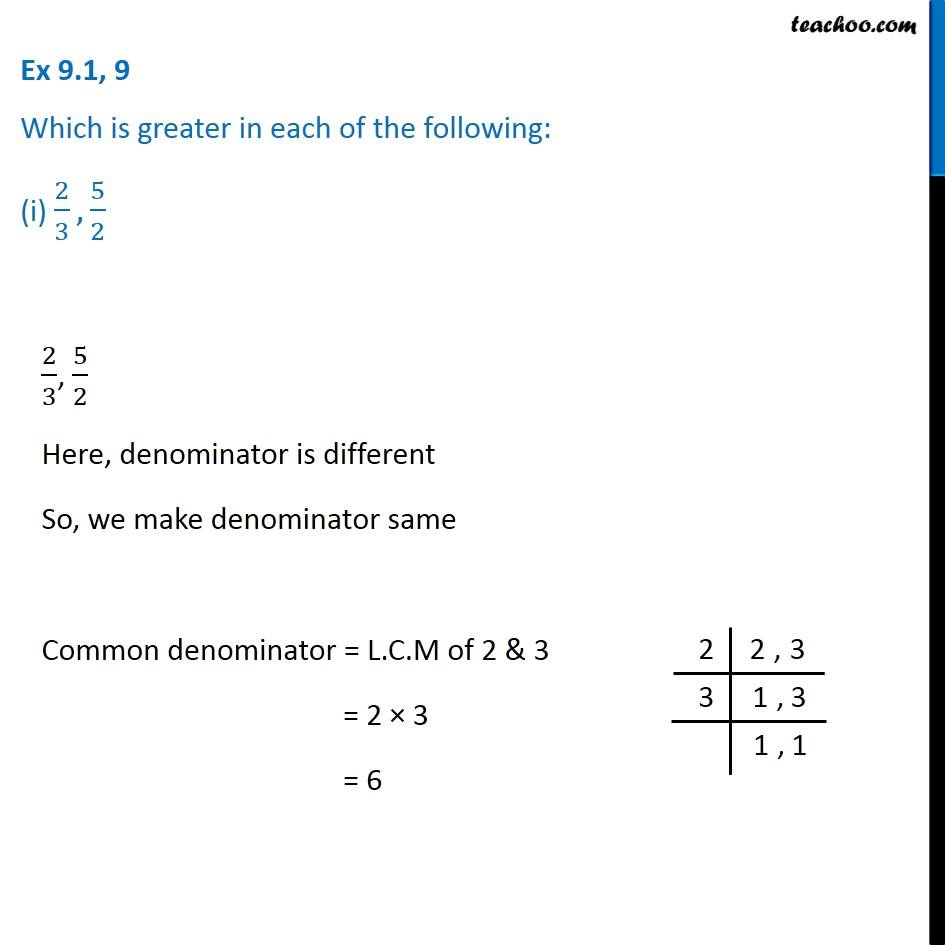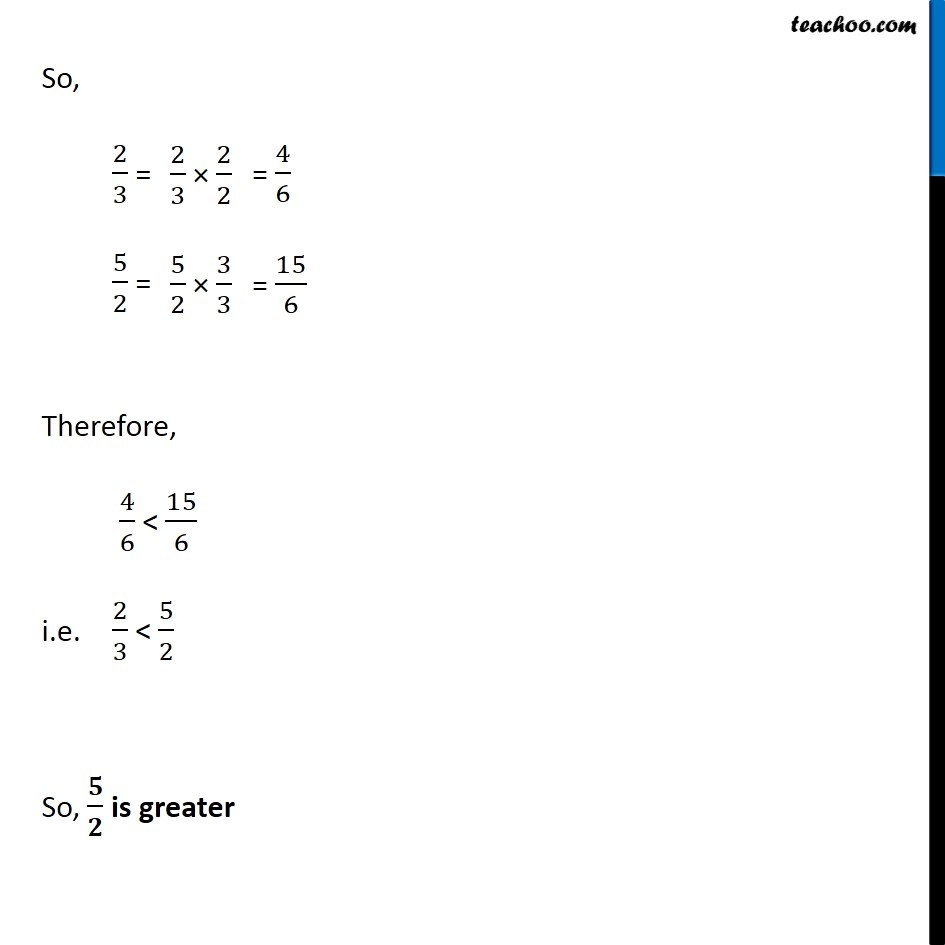Comparing rational numbers

Chapter 9 Class 7 Rational Numbers
Concept wiseIntroducing your new favourite teacher - Teachoo Black, at only ₹83 per month

### Transcript

Ex 9.1, 9 Which is greater in each of the following: (i) 2/3, 5/2 2/3, 5/2 Here, denominator is different So, we make denominator same Common denominator = L.C.M of 2 & 3 = 2 × 3 = 6 So, 2/3 = 5/2 = Therefore, 4/6 < 15/6 i.e. 2/3 < 5/2 So, 𝟓/𝟐 is greater